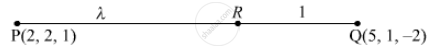Share

# The X-coordinate of a Point of the Line Joining the Points P(2,2,1) and Q(5,1,-2) is 4. Find Its Z-coordinate - CBSE (Commerce) Class 12 - Mathematics

ConceptVector and Cartesian Equation of a Plane

#### Question

The x-coordinate of a point of the line joining the points P(2,2,1) and Q(5,1,-2) is 4. Find its z-coordinate

#### Solution 1

Given P(2,2,1) and Q(5, 1,-2)

Let line divide PQ in the ratio k :1 and given x - coordinate of point on the line is 4 so by section formula

 k = (5k + 2)/(k+1)

4  = (5k + 2)/(k+1)

k = 2

Now, z-co-ordinate

z = (-2k+1)/(k+1) = (-2xx2+1)/(2+1) = -3/3 = -1

z = -1

z-coordinate = -1

#### Solution 2

Let the point R divide PQ in the ratio λ:1. Then, the coordinates of R will be (5lambda+2)/(lambda+1),(lambda+2)/(lambda+1), (-2lambda+1)/(lambda + 1)It is given that the x-coordinate of R is 4.

Therefore,

(5lambda+2)/(lambda+1) = 4

⇒ 5λ 4λ 4

⇒ 5λ − 4λ − 2

⇒ λ 2

Putting λ = 2 in (-2lambda + 1)/(lambda+1) we get

z-coordinate of R = (-2lambda + 1)/(lambda + 1) = (-2xx2xx1)/(2+1) = (-3)/3 = -1

Hence, the z-coordinate of the point is −1.

Is there an error in this question or solution?

#### Video TutorialsVIEW ALL 

Solution The X-coordinate of a Point of the Line Joining the Points P(2,2,1) and Q(5,1,-2) is 4. Find Its Z-coordinate Concept: Vector and Cartesian Equation of a Plane.
S